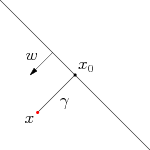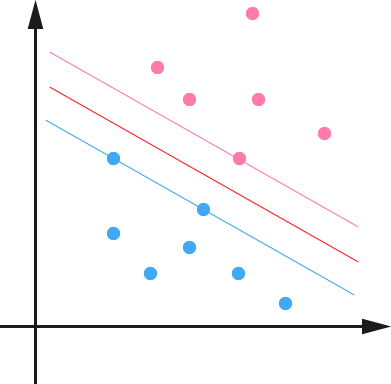SVM 一直被认为是效果最好的现成可用的分类算法之一（其实有很多人都相信，“之一”是可以去掉的）。这里“现成可用”其实是很重要的，因为一直以来学术界和工业界甚至只是学术界里做理论的和做应用的之间，都有一种“鸿沟”，有些很 fancy 或者很复杂的算法，在抽象出来的模型里很完美，然而在实际问题上却显得很脆弱，效果很差甚至完全 fail 。而 SVM 则正好是一个特例——在两边都混得开。

$$w^Tx + b = 0$$实际上这两个Criteria是互通的，我们定义 functional margin 为$$\hat{\gamma} = y(w^{T}x + b) = yf(x)$$，注意前面乘上类别$$y$$之后可以保证这个 margin 的非负性（因为$$f(x) < 0$$ 对应于$$y = -1$$的那些点），而点到超平面的距离定义为 geometrical margin 。不妨来看看二者之间的关系。如图所示，对于一个点$$x$$，令其垂直投影到超平面上的对应的为$$x_{0}$$，由于$$w$$是垂直于超平面的一个向量（请自行验证），我们有

$$x = x_{0} + \gamma\frac{w}{\|w\|}$$

$$\gamma = \frac{w^{T}x + b}{\|w\|} = \frac{f(x)}{\|w\|}$$

$$\tilde{\gamma} = y\gamma = \frac{\hat{\gamma}}{\|w\|}$$

$$\max \tilde{\gamma}$$

$$y_{i}(w^{T}x_{i} + b) = \hat{\gamma}_{i} \geq \hat{\gamma}, \quad i=1,\dots,n$$

$$\max \frac{1}{\|w\|}, \quad s.t., y_{i}(w^{T}x_{i} + b)\geq 1, i=1,\dots,n$$ps: 本文开头那张照片来源于这里。Allaboutinquiry 同学留言揭露典故真相啦： 😀